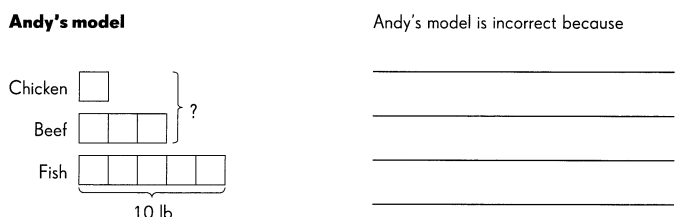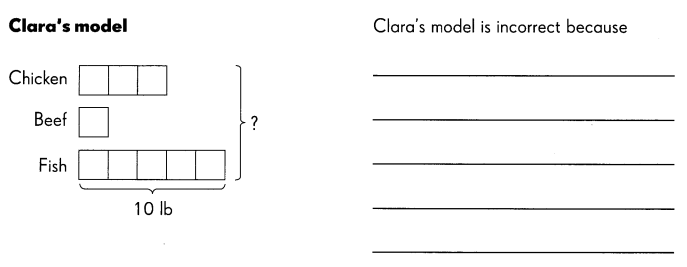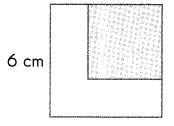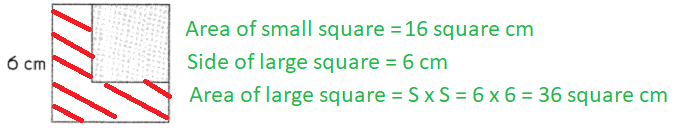Practice the problems of Math in Focus Grade 5 Workbook Answer Key Chapter 7 Ratio to score better marks in the exam.

Math Journal

Andy and Clara each drew a model to solve this word problem.

Mr. Marcos bought chicken and beef from the butcher and fish from the fish market for a barbecue. The ratio of the weight of chicken to the weight of beef to the weight of fish he bought was 3 : 1 : 5. He bought 10 pounds of fish.
What was the total weight of meat he bought from the butcher?

Both models however are incorrect.
Explain the mistakes that they each made.Draw the correct model. Then solve the problem.

8 pounds
Explanation:
Given,
Ratio of the weight of chicken to the weight of beef to the weight of fish is = 3 : 1 : 5
Mr. Marcos bought fish of = 10 pounds,
Total ratio = 3 + 1 + 5 = 9
Total pounds of chicken, beef and fish bought = X
Equation for pounds of fish bought = 5 / 9 x X = 10
=> X = 10 x 9 / 5
=> X = 2 x 9
=> X = 18
Pounds of chicken bought = 3 / 9 x 18 = 6 pounds
Pounds of beef bought = 1 / 9 x 18 = 2 pounds
Total weight of meat bought = 6 + 2 = 8 pounds
Therefore, Total weight of meat bought is 8 pounds.

Challenging Practice

Question 1.
A small square with an area of 16 square centimeters is cut from a larger square with sides that measure 6 centimeters. Find the ratio of the area of the small square to the area of the remaining part of the larger square.Explanation:
Given,
Area of small square = 16 square centimeters
Side of larger square = 6 centimeters
Area of small sqaure : Area of remaining part
16 square centimeters : ?
Area of remaining part = Area of large square – Area of small square
= 6 x 6 – 16
= 36 – 16
= 20 square centimeters
Ratio = area of small square : area of remaining part
= 16 : 20
= 4 : 5

Question 2.
The perimeters of two squares are in the ratio 2 : 4. The perimeter of the larger square is 16 centimeters.

a. What is the perimeter of the smaller square?
Answer: The perimeter of smaller square is 8 cm
Explanation:
Given,
The ratio of two squares are 2 : 4,
The perimeter of larger square is 16 centimeters,
By multiplying 2 and 4 with 4 we get the ratio 8 : 16,
Therefore, the perimeter of smaller square is 8 cm

b. What is the length of one side of the smaller square?
Answer: The length of one side of the smaller sqaure is 2 cm

Problem Solving

Solve.

Question 1.
The ratio of the number of plants Trish bought to the number of plants Sarah bought is 2 : 5. Trish bought 16 plants.
a. What is the total number of plants Trish and Sarah bought altogether?
Answer: The total number of plants Trish and Sarah bought are 56 plants
Explanation:
Given,
Ratio of plants Trish and Sarah bought are = 2 : 5,
Trish bought number of plants = 16,
By multiplying both the ratios 2 : 5 with 8, then we get 16 : 40,
By adding 16 with 40 we get 56,
Therefore, the total number of plants Trish and Sarah bought are 56 plants.

b. If each plant cost $1 7, what is the total cost of the plants Trish and Sarah bought? Answer: The total cost of the plants Trish and Sarah bought is$952.
Explanation:
Given,
Number of plants Trish and Sarah bought are = 56,
Each plant costs = $17, By multiplying 56 with 17 we get the sum as 952, Therefore, the total cost of 56 plants is$952.

Question 2.
The ratio of the number of boys to the number of girls at a town fair is 5 : 8 There are 60 boys at the fair.

a. What is the total number of boys and girls at the fair?
Answer:The total number of boys and girls at the fair is 156.
Explanation:
Given,
The ratio of number of boys to the number of girls = 5 : 8,
The number of boys at the fair = 60,
Total number of boys and girls = 5 : 8
By multiplying both 5 and 8 with 12 we get the ratio as 60 : 96,
Therefore, there are 60 boys and 96 girls,
By adding 60 with 96 we get the sum as 156.

b. The admission fee for each child is $3. Find the total admission fees for the boys and girls. Answer: The total admission fees for the boys and girls is$468.
Explanation:
Given,
Number of boys and girls = 156,
Admission fee for each child is = $3, By multiplying 156 with 3 we get the sum as 468, Therefore, the total admission fees for the boys and girls is$468.